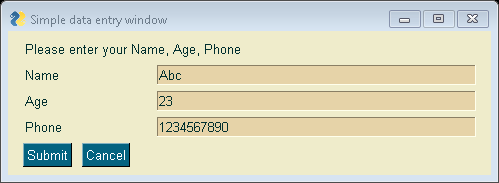Open In App

# User Input in PySimpleGUI

It is important how keys are key for understanding PySimpleGUI elements. If the user does not specify a key, then the element will be called an input element, a key will be provided to the user by default in integer form, starting the numbering with zero. If the user doesn’t specify any keys, then the values returned will be returned as a list as the keys are sequential integers.

This below example has no keys specified. The 3 input fields will have keys 0, 1, 2. Your first input element will be accessed as values, value for the second, value for the third, and so on.

Example: Python Program for User Input using PySimpleGUI .

## Python3

 `import` `PySimpleGUI as sg`` ` `# Add some color``# to the window``sg.theme(``'SandyBeach'``)     `` ` `# Very basic window.``# Return values using``# automatic-numbered keys``layout ``=` `[``    ``[sg.Text(``'Please enter your Name, Age, Phone'``)],``    ``[sg.Text(``'Name'``, size ``=``(``15``, ``1``)), sg.InputText()],``    ``[sg.Text(``'Age'``, size ``=``(``15``, ``1``)), sg.InputText()],``    ``[sg.Text(``'Phone'``, size ``=``(``15``, ``1``)), sg.InputText()],``    ``[sg.Submit(), sg.Cancel()]``]`` ` `window ``=` `sg.Window(``'Simple data entry window'``, layout)``event, values ``=` `window.read()``window.close()`` ` `# The input data looks like a simple list ``# when automatic numbered``print``(event, values[``0``], values[``1``], values[``2``])   `

Output: